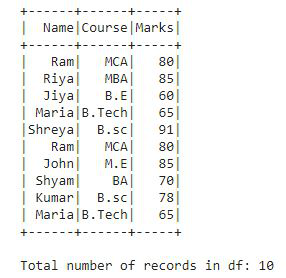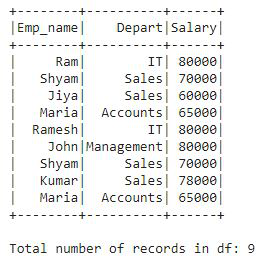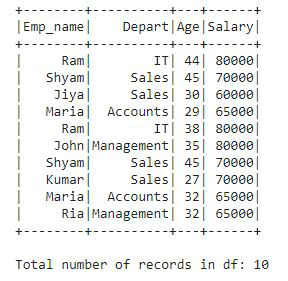Related Articles

# PySpark Count Distinct from DataFrame

• Last Updated : 23 May, 2021

In this article, we will discuss how to count distinct values present in the Pyspark DataFrame.

In Pyspark, there are two ways to get the count of distinct values. We can use distinct() and count() functions of DataFrame to get the count distinct of PySpark DataFrame. Another way is to use SQL countDistinct() function which will provide the distinct value count of all the selected columns. Let’s understand both the ways to count distinct from DataFrame with examples.

### Method 1: distinct().count():

The distinct and count are the two different functions that can be applied to DataFrames. distinct() will eliminate all the duplicate values or records by checking all columns of a Row from DataFrame and count() will return the count of records on DataFrame. By chaining these two functions one after the other we can get the count distinct of PySpark DataFrame.

Example 1: Pyspark Count Distinct from DataFrame using distinct().count()

In this example, we will create a DataFrame df which contains Student details like Name, Course, and Marks. The DataFrame contains some duplicate values also. And we will apply the distinct().count() to find out all the distinct values count present in the DataFrame df.

## Python3

 `# importing module``import` `pyspark`` ` `# importing sparksession from ``# pyspark.sql mudule``from` `pyspark.sql ``import` `SparkSession`` ` `# creating sparksession and giving``# app name``spark ``=` `SparkSession.builder.appName(``'sparkdf'``).getOrCreate()`` ` `# giving rows value for dataframe``data ``=` `[(``"Ram"``, ``"MCA"``, ``80``),``        ``(``"Riya"``, ``"MBA"``, ``85``),``        ``(``"Jiya"``, ``"B.E"``, ``60``),``        ``(``"Maria"``, ``"B.Tech"``, ``65``),``        ``(``"Shreya"``, ``"B.sc"``, ``91``),``        ``(``"Ram"``, ``"MCA"``, ``80``),``        ``(``"John"``, ``"M.E"``, ``85``),``        ``(``"Shyam"``, ``"BA"``, ``70``),``        ``(``"Kumar"``, ``"B.sc"``, ``78``),``        ``(``"Maria"``, ``"B.Tech"``, ``65``)]`` ` `# giving column names of dataframe``columns ``=` `[``"Name"``, ``"Course"``, ``"Marks"``]`` ` `# creating a dataframe df``df ``=` `spark.createDataFrame(data, columns)`` ` `# show df``df.show()`` ` `# counting the total number of values ``# in df``print``(``"Total number of records in df:"``, df.count())`

Output:This is the DataFrame df that we have created, and it contains total of 10 records. Now, we apply distinct().count() to find out the total distinct value count present in the DataFrame df.

## Python3

 `# applying distinct().count() on df``print``(``'Distinct count in DataFrame df is :'``, df.distinct().count())`

Output:

`Distinct count in DataFrame df is : 8`

In this output, we can see that there are 8 distinct values present in the DataFrame df.

### Method 2: countDistinct():

This function provides the count of distinct elements present in a group of selected columns. countDistinct() is an SQL function that will provide the distinct value count of all the selected columns.

Example 1: Pyspark Count Distinct from DataFrame using countDistinct().

In this example, we will create a DataFrame df that contains employee details like Emp_name, Department, and Salary. The DataFrame contains some duplicate values also. And we will apply the countDistinct() to find out all the distinct values count present in the DataFrame df.

## Python3

 `# importing sparksession from ``# pyspark.sql mudule``from` `pyspark.sql ``import` `SparkSession`` ` `# creating sparksession and giving ``# app name``spark ``=` `SparkSession.builder.appName(``'sparkdf'``).getOrCreate()`` ` `# giving rows value for dataframe``data ``=` `[(``"Ram"``, ``"IT"``, ``80000``),``        ``(``"Shyam"``, ``"Sales"``, ``70000``),``        ``(``"Jiya"``, ``"Sales"``, ``60000``),``        ``(``"Maria"``, ``"Accounts"``, ``65000``),``        ``(``"Ramesh"``, ``"IT"``, ``80000``),``        ``(``"John"``, ``"Management"``, ``80000``),``        ``(``"Shyam"``, ``"Sales"``, ``70000``),``        ``(``"Kumar"``, ``"Sales"``, ``78000``),``        ``(``"Maria"``, ``"Accounts"``, ``65000``)]`` ` `# giving column names of dataframe``columns ``=` `[``"Emp_name"``, ``"Depart"``, ``"Salary"``]`` ` `# creating a dataframe df``df ``=` `spark.createDataFrame(data, columns)`` ` `# show df``df.show()`` ` `# counting the total number of values in df``print``(``"Total number of records in df:"``, df.count())`This is the DataFrame df that we have created, and it contains total of 9 records. Now, we will apply countDistinct() to find out the total distinct value count present in the DataFrame df. To apply this function we will import the function from pyspark.sql.functions module.

## Python3

 `# importing countDistinct from``# pyspark.sql.functions``from` `pyspark.sql.functions ``import` `countDistinct`` ` `# applying the function countDistinct() ``# on df using select()``df2 ``=` `df.select(countDistinct(``"Emp_name"``, ``"Depart"``, ``"Salary"``))`` ` `# show df2``df2.show()`

Output:

```+----------------------------------------+
|count(DISTINCT Emp_name, Depart, Salary)|
+----------------------------------------+
|                                       7|
+----------------------------------------+```

There are 7 distinct records present in DataFrame df. The countDistinct() provides the distinct count value in the column format as shown in the output as it’s an SQL function.

Now, let’s see the distinct values count based on one particular column. We will count the distinct values present in the Department column of employee details df.

## Python3

 `# importing countDistinct from ``# pyspark.sql.functions``from` `pyspark.sql.functions ``import` `countDistinct`` ` `# applying the function countDistinct() ``# on df using select()``df3 ``=` `df.select(countDistinct(``"Depart"``))`` ` `# show df2``df3.show()`

Output:

```+----------------------+
|count(DISTINCT Depart)|
+----------------------+
|                     4|
+----------------------+```

There are 4 distinct values present in the department column. In this example, we have applied countDistinct() only on Depart column.

Example 2: Pyspark Count Distinct from DataFrame using SQL query.

In this example, we have created a dataframe containing employee details like Emp_name, Depart, Age, and Salary. Now, we will count the distinct records in the dataframe using a simple SQL query as we use in SQL. Let’s see the example and understand it:

## Python3

 `# importing sparksession from pyspark.sql mudule``from` `pyspark.sql ``import` `SparkSession`` ` `# creating sparksession and giving app name``spark ``=` `SparkSession.builder.appName(``'sparkdf'``).getOrCreate()`` ` `# giving rows value for dataframe``data ``=` `[(``"Ram"``, ``"IT"``, ``44``, ``80000``),``        ``(``"Shyam"``, ``"Sales"``, ``45``, ``70000``),``        ``(``"Jiya"``, ``"Sales"``, ``30``, ``60000``),``        ``(``"Maria"``, ``"Accounts"``, ``29``, ``65000``),``        ``(``"Ram"``, ``"IT"``, ``38``, ``80000``),``        ``(``"John"``, ``"Management"``, ``35``, ``80000``),``        ``(``"Shyam"``, ``"Sales"``, ``45``, ``70000``),``        ``(``"Kumar"``, ``"Sales"``, ``27``, ``70000``),``        ``(``"Maria"``, ``"Accounts"``, ``32``, ``65000``),``        ``(``"Ria"``, ``"Management"``, ``32``, ``65000``)]`` ` `# giving column names of dataframe``columns ``=` `[``"Emp_name"``, ``"Depart"``, ``"Age"``, ``"Salary"``]`` ` `# creating a dataframe df``df ``=` `spark.createDataFrame(data, columns)`` ` `# show df``df.show()`` ` `# counting the total number of values in df``print``(``"Total number of records in df:"``, df.count())`

Output:This is the dataframe that contains total of 10 records along with some duplicate records also. Now, we will use an SQL query and find out how many distinct records are found in this dataframe. It is as simple as we do in SQL.

## Python3

 `# creating a temporary view of ``# Dataframe and storing it into df2``df.createOrReplaceTempView(``"df2"``)`` ` `# using the SQL query to count all``# distinct records and display the``# count on the screen``spark.sql(``"select count(distinct(*)) from df2"``).show()`

#### Output:

```+---------------------------------------------+
|count(DISTINCT Emp_name, Depart, Age, Salary)|
+---------------------------------------------+
|                                            9|
+---------------------------------------------+```

There are 9 distinct records found in the entire dataframe df.

Now let’s find the distinct values count in two columns i.e. Emp_name and Salary using the below SQL query.

## Python3

 `# using the SQL query to count distinct``# records in 2 columns only display the``# count on the screen``spark.sql(``"select count(distinct(Emp_name, Salary)) from df2"``).show()`

Output:

```+----------------------------------------------------------------+
|count(DISTINCT named_struct(Emp_name, Emp_name, Salary, Salary))|
+----------------------------------------------------------------+
|                                                               7|
+----------------------------------------------------------------+```

There are 7 distinct values found in Emp_name and Salary column.

As SQL provides the output of all the operations performed on the data in the tabular format. We got the answer in the column that contains two rows, the first row has the heading, and second row contains a distinct count of records. In Example2 also got output in the same format as, countDistinct() is also an SQL function.

Attention geek! Strengthen your foundations with the Python Programming Foundation Course and learn the basics.

To begin with, your interview preparations Enhance your Data Structures concepts with the Python DS Course. And to begin with your Machine Learning Journey, join the Machine Learning – Basic Level Course

My Personal Notes arrow_drop_up